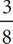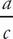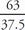# SAT Math Multiple Choice Question 27: Answer and Explanation

### Test Information

Question: 27

3. If a is 63% of x and c isof x, which of the following is the closest equivalent of the ratio of a to c ?

• A. 0.236
• B. 0.381
• C. 0.595
• D. 1.68

D Since you are never told what x is, and there is no way to find it, plug in for x. Say that x = 100. 63% of 100 is 63, andof 100 is 37.5. The ratio of a to c is. So,= 1.68. To save time, you can ballpark the answer, since a > c and (D) is the only choice greater than 1.